# Diagonal continued fractionin which the sequences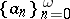andmust satisfy the following conditions:

1) the numbersandare integers;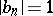;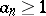if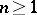;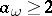if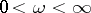;

2)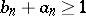for all; if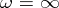, then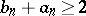for an infinite set of indices;

3)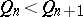for all;

4) the partial fractions of the continued fraction are all irreducible fractionssuch that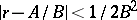and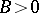, whereis value of the continued fraction.

For each real numberthere exists one and only one diagonal continued fraction withas its value; this fraction is periodic ifis a quadratic irrationality.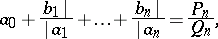with,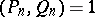,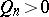. These are the numbers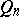alluded to in condition . The continued fraction as described above for a real numbercan be obtained by the nearest integer algorithm, that is,,,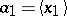,,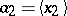, etc., where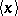denotes the nearest integer to. It is also possible to use the entier function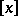instead of. One then has the continued fraction algorithm which is more commonly used.
The adjective "diagonal" stems from the fact that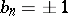for all.